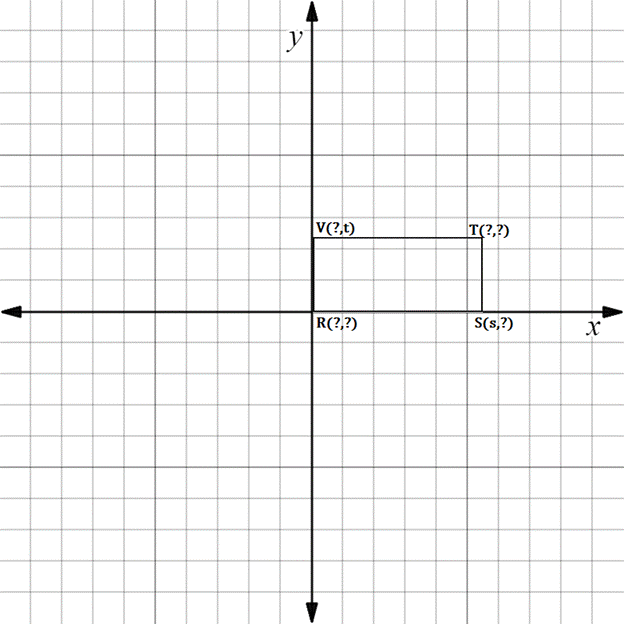Chapter 10.3, Problem 16E### Elementary Geometry for College St...

6th Edition
Daniel C. Alexander + 1 other
ISBN: 9781285195698

#### Solutions

Chapter
Section### Elementary Geometry for College St...

6th Edition
Daniel C. Alexander + 1 other
ISBN: 9781285195698
Textbook Problem
21 views

# In Exercises 11 to 16, supply the missing coordinates for the vertices, using as few variables as possible.R S T V is a rectangle.

To determine

To find:

The missing coordinates for the vertices.

Explanation

The given figure is shown below.

From the figure, the following observations are made.

Since, the vertex R is at the origin.

The coordinates of R0, 0.

Now, consider the vertex S.

Here, x-coordinate is given as s.

In the S vertex, observe where it lies on y-axis.

Since, it is at 0, the vertex S has the coordinates s, 0.

Consider the vertex V.

Here, y-coordinate is t.

x-coordinate is 0.

The vertex V has coordinates 0, t.

The distance from R to S is s

### Still sussing out bartleby?

Check out a sample textbook solution.

See a sample solution

#### The Solution to Your Study Problems

Bartleby provides explanations to thousands of textbook problems written by our experts, many with advanced degrees!

Get Started